## Learn The Addition Rule Of Probability And Adding Probabilities With Example Problems & Interactive Exercises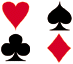Experiment: A single 6-sided die is rolled. What is the probability of rolling a 2 or a 5?Possibilities:

1. The number rolled can be a 2.

2. The number rolled can be a 5.

Events: These events are mutually exclusive since they cannot occur at the same time.

Probabilities: How do we find the probabilities of these mutually exclusive events? We need a rule to guide us.

Addition Rule 1: When two events, A and B, are mutually exclusive, the probability that A or B will occur is the sum of the probability of each event.

P(A or B) = P(A) + P(B)

Let's use this addition rule to find the probability for Experiment 1.

Experiment 1: A single 6-sided die is rolled. What is the probability of rolling a 2 or a 5?

Probabilities:

 P(2) = 1 6P(5) = 1 6P(2 or 5) = P(2) + P(5)= 1 + 1 6 6= 2 6= 1 3

Experiment 2: A spinner has 4 equal sectors colored yellow, blue, green, and red. What is the probability of landing on red or blue after spinning this spinner?Probabilities:

 P(red) = 1 4P(blue) = 1 4P(red or blue) = P(red) + P(blue)= 1 + 1 4 4= 2 4= 1 2Experiment 3: A glass jar contains 1 red, 3 green, 2 blue, and 4 yellow marbles. If a single marble is chosen at random from the jar, what is the probability that it is yellow or green?

Probabilities:

 P(yellow) = 4 10P(green) = 3 10P(yellow or green) = P(yellow) + P(green)= 4 + 3 10 10= 7 10

In each of the three experiments above, the events are mutually exclusive. Let's look at some experiments in which the events are non-mutually exclusive.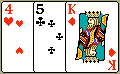Experiment 4: A single card is chosen at random from a standard deck of 52 playing cards. What is the probability of choosing a king or a club?

Probabilities:

 P(king or club) = P(king) + P(club) - P(king of clubs)= 4 + 13 - 1 52 52 52= 16 52= 4 13

In Experiment 4, the events are non-mutually exclusive. The addition causes the king of clubs to be counted twice, so its probability must be subtracted. When two events are non-mutually exclusive, a different addition rule must be used.

Additional Rule 2: When two events, A and B, are non-mutually exclusive, the probability that A or B will occur is:

P(A or B) = P(A) + P(B) - P(A and B)

In the rule above, P(A and B) refers to the overlap of the two events. Let's apply this rule to some other experiments.Experiment 5: In a math class of 30 students, 17 are boys and 13 are girls. On a unit test, 4 boys and 5 girls made an A grade. If a student is chosen at random from the class, what is the probability of choosing a girl or an A student?

Probabilities: P(girl or A) = P(girl) + P(A) - P(girl and A)

 = 13 + 9 - 5 30 30 30= 17 30Experiment 6: On New Year's Eve, the probability of a person having a car accident is 0.09. The probability of a person driving while intoxicated is 0.32 and probability of a person having a car accident while intoxicated is 0.15. What is the probability of a person driving while intoxicated or having a car accident?

Probabilities:

 P(intoxicated or accident) = P(intoxicated) + P(accident) - P(intoxicated and accident)= 0.32 + 0.09 - 0.15 = 0.26

Summary: To find the probability of event A or B, we must first determine whether the events are mutually exclusive or non-mutually exclusive. Then we can apply the appropriate Addition Rule:

Addition Rule 1: When two events, A and B, are mutually exclusive, the probability that A or B will occur is the sum of the probability of each event.

P(A or B) = P(A) + P(B)

Addition Rule 2: When two events, A and B, are non-mutually exclusive, there is some overlap between these events. The probability that A or B will occur is the sum of the probability of each event, minus the probability of the overlap.

P(A or B) = P(A) + P(B) - P(A and B)

### Exercises

Directions: Read each question below. Select your answer by clicking on its button. Feedback to your answer is provided in the RESULTS BOX. If you make a mistake, choose a different button.

 1. A day of the week is chosen at random. What is the probability of choosing a Monday or Tuesday?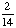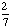None of the above. RESULTS BOX:
 2. In a pet store, there are 6 puppies, 9 kittens, 4 gerbils and 7 parakeets. If a pet is chosen at random, what is the probability of choosing a puppy or a parakeet? 1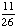None of the above. RESULTS BOX:
 3. The probability of a New York teenager owning a skateboard is 0.37, of owning a bicycle is 0.81 and of owning both is 0.36. If a New York teenager is chosen at random, what is the probability that the teenager owns a skateboard or a bicycle? 1.180.70.82None of the above. RESULTS BOX:
 4. A number from 1 to 10 is chosen at random. What is the probability of choosing a 5 or an even number?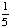All of the above. RESULTS BOX:
 5. A single 6-sided die is rolled. What is the probability of rolling a number greater than 3 or an even number? 1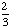None of the above. RESULTS BOX: